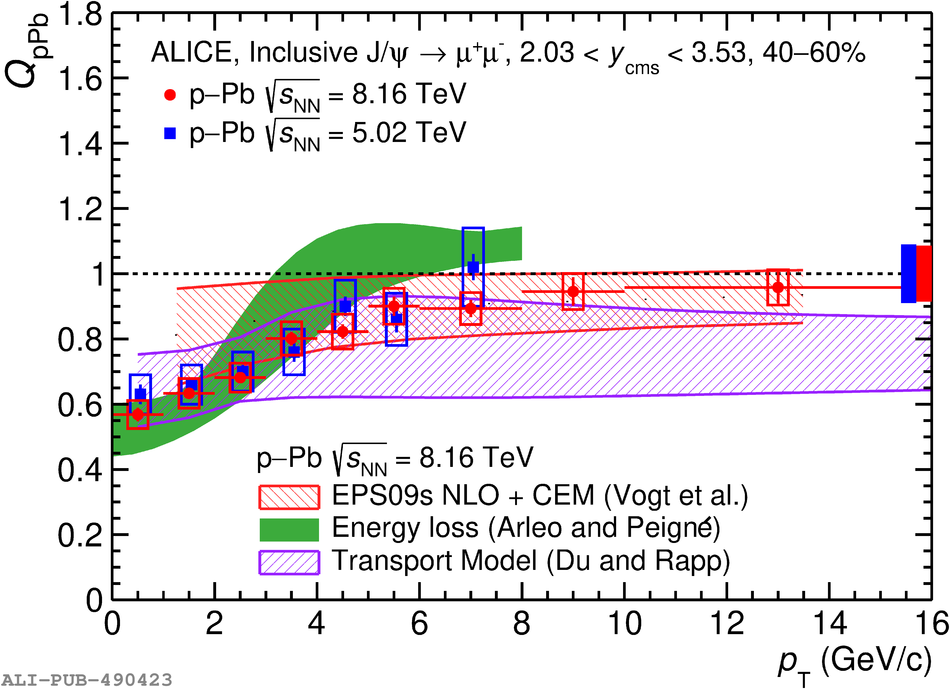# Figure 8

 Inclusive J/$\psi$ $Q_{\rm pPb}$ as a function of $p_{\rm T}$ for 2--10\%, 10--20\%, 20--40\%, 40--60\%, 60--80\%, and 80--90\% ZN centrality classes at forward rapidity in p--Pb collisions at $\sqrt{s_{\rm NN}} = 8.16$ TeV compared with the results at $\sqrt{s_{\rm NN}} = 5.02$ TeV  and with theoretical calculations . The vertical error bars show the statistical uncertainties, the open boxes the uncorrelated systematic uncertainties, and the full boxes centered at $Q_{\rm pPb} = 1$ the correlated systematic uncertainties.